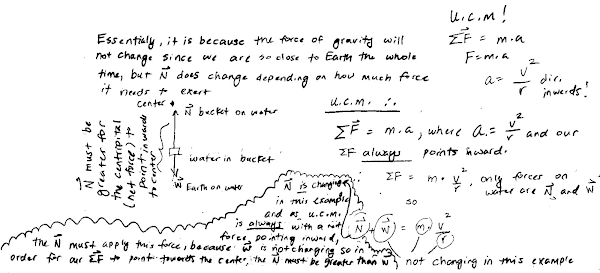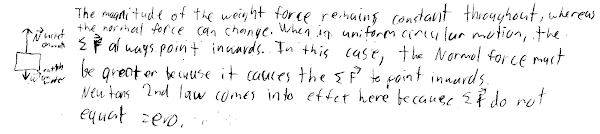## 20141021

### Physics midterm question: water-filled bucket twirled in a vertical circle

Physics 205A Midterm 1, fall semester 2014
Cuesta College, San Luis Obispo, CA

Cf. Giambattista/Richardson/Richardson, Physics, 2/e, Problem 5.39Water is poured into a bucket attached to an ideal string, and is then twirled around in a circle. Discuss why the magnitude of the normal force of the bucket on the water is greater than the magnitude of the weight force of Earth on the water, when the bucket is at the bottom of its circular path. Explain your reasoning by using a free-body diagram, the properties of forces and Newton's laws.

• p:
Correct. Understands that the water in the bucket has two vertical forces acting on it:
Weight force of Earth on water (downwards, magnitude w = m·g).
Normal force of bucket on water (upwards, magnitude varies but must be greater than w).
Because the water is (momentarily) undergoing uniform circular motion as it goes through the bottom of its swing, then Newton's second law applies to the motion of the water (speed is constant but direction of the velocity vector is changing), and the net force must point upwards (towards the center of the circular path). This means that the upwards normal force must have a magnitude greater than the magnitude of the weight force.
• r:
As (p), but argument indirectly, weakly, or only by definition supports the statement to be proven, or has minor inconsistencies or loopholes. Some attempt at applying Newton's laws to a free-body diagram, but may have a centrifugal or centripetal "m·v/r2" force in addition to the normal and weight forces.
• t:
Nearly correct, but argument has conceptual errors, or is incomplete. Problematic attempt at applying Newton's laws (N1 or N3) to a free-body diagram (with extraneous or missing forces, or inconsistent with Newton's laws).
• v:
Limited relevant discussion of supporting evidence of at least some merit, but in an inconsistent or unclear manner. Effectively only has a substantive attempt at a free-body diagram, or only a substantive attempt at discussing Newton's laws.
• x:
Implementation of ideas, but credit given for effort rather than merit. Approach other than that of applying Newton's laws to a free-body diagram.
• y:
Irrelevant discussion/effectively blank.
• z:
Blank.
Sections 70854, 70855, 73320
Exam code: midterm01m0oU
p: 8 students
r: 24 students
t: 28 students
v: 13 students
x: 0 students
y: 0 students
z: 1 student

A sample "p" response (from student 0203):Another sample "p" response (from student 1828):A sample "r" response (from student 1914), with an additional centripetal force on the free-body diagram:A sample "v" response (from student 3474):